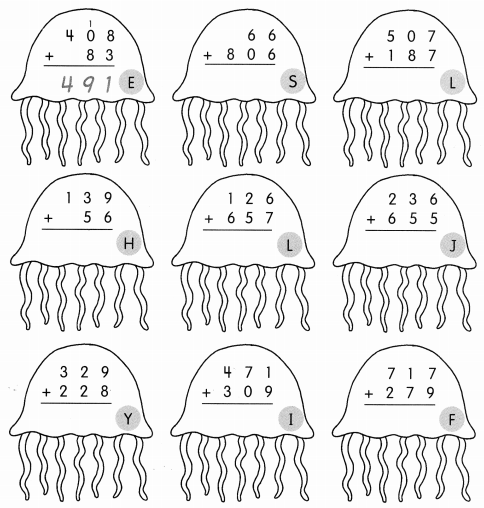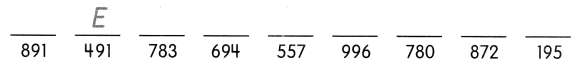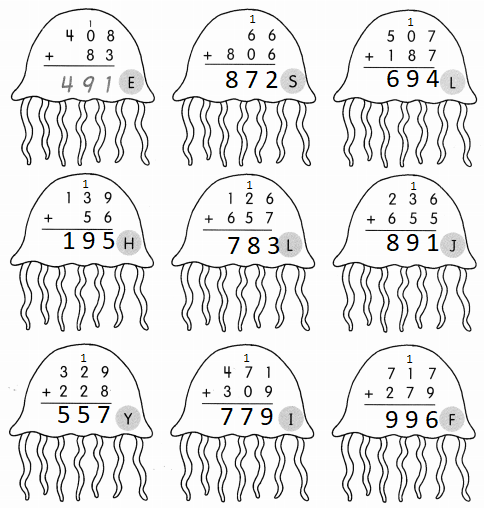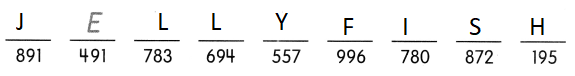# Math in Focus Grade 2 Chapter 2 Practice 3 Answer Key Addition with Regrouping in Ones

Go through the Math in Focus Grade 2 Workbook Answer Key Chapter 2 Practice 3 Addition with Regrouping in Ones to finish your assignments.

## Math in Focus Grade 2 Chapter 2 Practice 3 Answer Key Addition with Regrouping in Ones

Question 1.
778 + 119 = ?
8 ones + 9 ones = 17 ones 8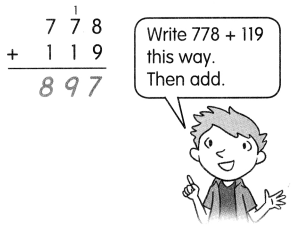= _________ ten _________ ones
1 ten + 7 tens + 1 ten = _________ tens
7 hundreds + 1 hundred = _________ hundreds
778 + 119 = ______
17 ones = 1 ten 7 ones
1 ten + 7 tens + 1 ten = 9 tens
7 hundreds + 1 hundred = 8 hundreds
778 + 119 = 897

Question 2.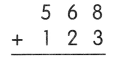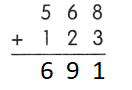Explanation:
8 ones and 3 ones = 11 ones =  1 ten 1 ones
1 ten + 6 tens + 2 ten = 9 tens
5 hundreds + 1 hundred = 6 hundreds
568 + 123 = 691

Question 3.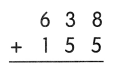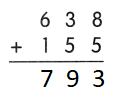Explanation:
8 ones + 5 ones  = 13 ones =  1 ten 3 ones
1 ten + 3 tens + 5 ten = 9 tens
6 hundreds + 1 hundred = 7 hundreds
638 + 155 = 793

Question 4.
576 + 207 = ___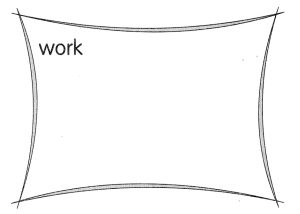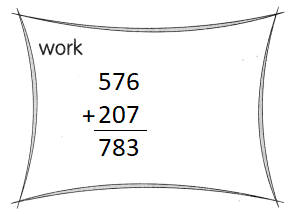Explanation:
The sum of 576 + 207 = 783

Question 5.
631 + 329 = ___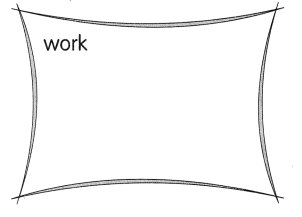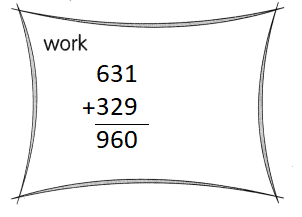Explanation:
The sum of 631 + 329 = 960